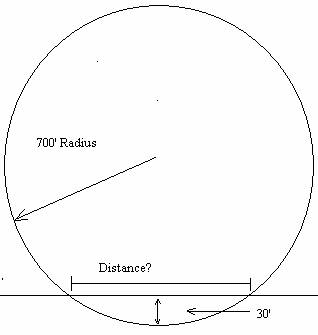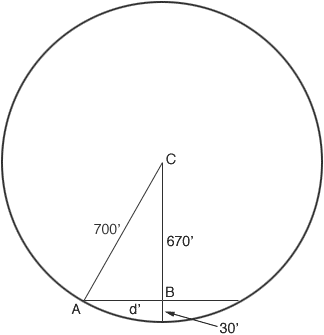Quandaries and Queries Hi, my name is Bruce, I would like to solve the following problem illustrated below. How do you calculate the length of a line that intersects a circle. Obviously I shouldn't’t sleep in geometry class. Hi Bruce, I reproduced the diagram you sentand drew it again with some points labeled so that I can talk about them.C is the centre of the circle, A is one endpoint of your line segment and B is the midpoint of the line segment. Let d be the length of AB then d is half the length of your line segment. Triangle ABC is a right triangle. Use Pythagoras theorem on this triangle and solve the resulting equation for d. Penny